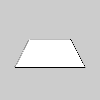# rotate_x()#

Rotates around the x-axis the amount specified by the angle parameter.

## Examples#def setup():
py5.size(100, 100, py5.P3D)
py5.translate(py5.width//2, py5.height//2)
py5.rotate_x(py5.PI/3.0)
py5.rect(-26, -26, 52, 52)def setup():
py5.size(100, 100, py5.P3D)
py5.translate(py5.width//2, py5.height//2)
py5.rect(-26, -26, 52, 52)


## Description#

Rotates around the x-axis the amount specified by the angle parameter. Angles should be specified in radians (values from 0 to TWO_PI) or converted from degrees to radians with the radians() function. Coordinates are always rotated around their relative position to the origin. Positive numbers rotate in a clockwise direction and negative numbers rotate in a counterclockwise direction. Transformations apply to everything that happens after and subsequent calls to the function accumulates the effect. For example, calling rotate_x(PI/2) and then rotate_x(PI/2) is the same as rotate_x(PI). If rotate_x() is run within the draw(), the transformation is reset when the loop begins again. This function requires using P3D as a third parameter to size() as shown in the example.

Underlying Processing method: rotateX

## Signatures#

rotate_x(
angle: float,  # angle of rotation specified in radians
/,
) -> None


Updated on March 06, 2023 02:49:26am UTC